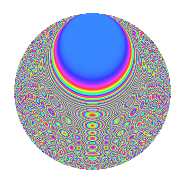# Properties

 Label 1575.2.bxLevel 1575 Weight 2 Character orbit bx Rep. character $$\chi_{1575}(64,\cdot)$$ Character field $$\Q(\zeta_{10})$$ Dimension 296 Sturm bound 480

# Learn more about

## Defining parameters

 Level: $$N$$ = $$1575 = 3^{2} \cdot 5^{2} \cdot 7$$ Weight: $$k$$ = $$2$$ Character orbit: $$[\chi]$$ = 1575.bx (of order $$10$$ and degree $$4$$) Character conductor: $$\operatorname{cond}(\chi)$$ = $$25$$ Character field: $$\Q(\zeta_{10})$$ Sturm bound: $$480$$

## Dimensions

The following table gives the dimensions of various subspaces of $$M_{2}(1575, [\chi])$$.

Total New Old
Modular forms 992 296 696
Cusp forms 928 296 632
Eisenstein series 64 0 64

## Trace form

 $$296q$$ $$\mathstrut +\mathstrut 72q^{4}$$ $$\mathstrut +\mathstrut 10q^{5}$$ $$\mathstrut +\mathstrut O(q^{10})$$ $$296q$$ $$\mathstrut +\mathstrut 72q^{4}$$ $$\mathstrut +\mathstrut 10q^{5}$$ $$\mathstrut +\mathstrut 8q^{10}$$ $$\mathstrut -\mathstrut 8q^{11}$$ $$\mathstrut +\mathstrut 4q^{14}$$ $$\mathstrut -\mathstrut 44q^{16}$$ $$\mathstrut -\mathstrut 12q^{19}$$ $$\mathstrut -\mathstrut 70q^{22}$$ $$\mathstrut +\mathstrut 10q^{23}$$ $$\mathstrut +\mathstrut 40q^{25}$$ $$\mathstrut +\mathstrut 12q^{26}$$ $$\mathstrut +\mathstrut 22q^{29}$$ $$\mathstrut +\mathstrut 12q^{31}$$ $$\mathstrut -\mathstrut 12q^{34}$$ $$\mathstrut +\mathstrut 2q^{35}$$ $$\mathstrut -\mathstrut 10q^{37}$$ $$\mathstrut +\mathstrut 70q^{38}$$ $$\mathstrut +\mathstrut 126q^{40}$$ $$\mathstrut +\mathstrut 4q^{41}$$ $$\mathstrut +\mathstrut 62q^{44}$$ $$\mathstrut +\mathstrut 20q^{46}$$ $$\mathstrut +\mathstrut 90q^{47}$$ $$\mathstrut -\mathstrut 296q^{49}$$ $$\mathstrut -\mathstrut 94q^{50}$$ $$\mathstrut -\mathstrut 20q^{53}$$ $$\mathstrut +\mathstrut 6q^{55}$$ $$\mathstrut -\mathstrut 12q^{56}$$ $$\mathstrut +\mathstrut 130q^{58}$$ $$\mathstrut -\mathstrut 18q^{59}$$ $$\mathstrut +\mathstrut 20q^{61}$$ $$\mathstrut +\mathstrut 50q^{62}$$ $$\mathstrut +\mathstrut 36q^{64}$$ $$\mathstrut -\mathstrut 98q^{65}$$ $$\mathstrut -\mathstrut 70q^{67}$$ $$\mathstrut -\mathstrut 8q^{70}$$ $$\mathstrut -\mathstrut 24q^{71}$$ $$\mathstrut -\mathstrut 40q^{73}$$ $$\mathstrut -\mathstrut 44q^{74}$$ $$\mathstrut -\mathstrut 12q^{76}$$ $$\mathstrut -\mathstrut 20q^{77}$$ $$\mathstrut -\mathstrut 48q^{79}$$ $$\mathstrut -\mathstrut 80q^{80}$$ $$\mathstrut -\mathstrut 30q^{83}$$ $$\mathstrut -\mathstrut 52q^{85}$$ $$\mathstrut -\mathstrut 100q^{86}$$ $$\mathstrut -\mathstrut 140q^{88}$$ $$\mathstrut -\mathstrut 2q^{89}$$ $$\mathstrut +\mathstrut 8q^{91}$$ $$\mathstrut +\mathstrut 120q^{92}$$ $$\mathstrut -\mathstrut 54q^{95}$$ $$\mathstrut -\mathstrut 30q^{97}$$ $$\mathstrut +\mathstrut O(q^{100})$$

## Decomposition of $$S_{2}^{\mathrm{new}}(1575, [\chi])$$ into irreducible Hecke orbits

The newforms in this space have not yet been added to the LMFDB.

## Decomposition of $$S_{2}^{\mathrm{old}}(1575, [\chi])$$ into lower level spaces

$$S_{2}^{\mathrm{old}}(1575, [\chi]) \cong$$ $$S_{2}^{\mathrm{new}}(25, [\chi])$$$$^{\oplus 6}$$$$\oplus$$$$S_{2}^{\mathrm{new}}(75, [\chi])$$$$^{\oplus 4}$$$$\oplus$$$$S_{2}^{\mathrm{new}}(175, [\chi])$$$$^{\oplus 3}$$$$\oplus$$$$S_{2}^{\mathrm{new}}(225, [\chi])$$$$^{\oplus 2}$$$$\oplus$$$$S_{2}^{\mathrm{new}}(525, [\chi])$$$$^{\oplus 2}$$